06按钮，选择支和开始界面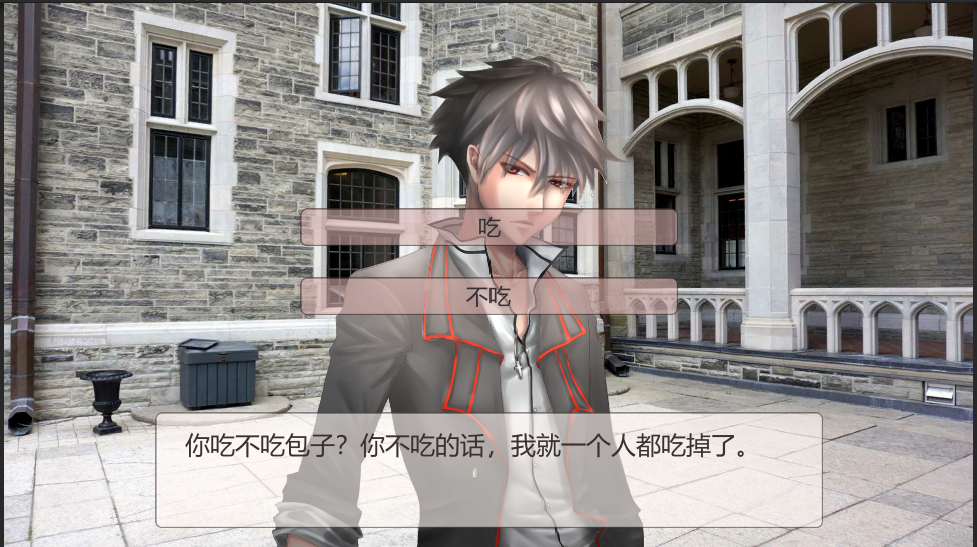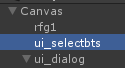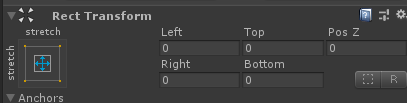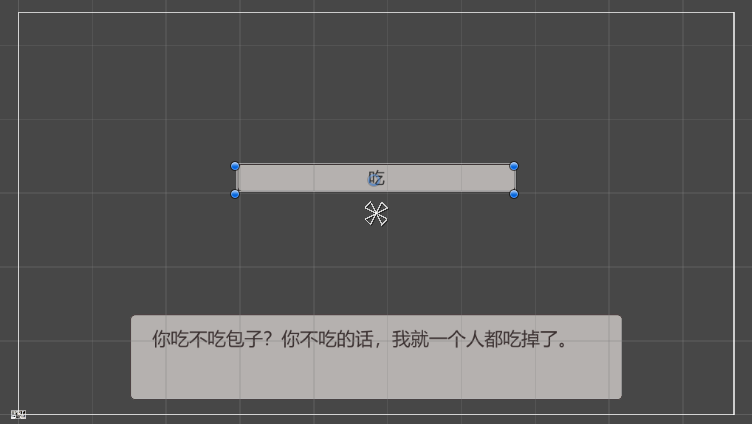button会自带一个text的子object，如果你的按钮是图片上写死了文字的那种，可以把这个text删掉，但是我们现在是在做选择支，上边的文字必然是由脚本决定的，所以这个text要留着，为了测试效果，我们把它默认的文字改成“吃”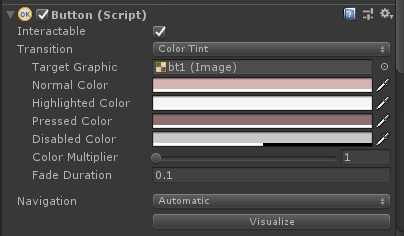animation模式是切换录制的动画，鉴于我们之前多次做过录制动画的部分，如果想要在这里使用应该对于现在的你也是很容易的。可以用来做非常豪华的按钮。

none就是完全没有变化，虽然还是会响应点击事件，但是画面上不会看出来有什么改变。bt1已经没什么问题了，我们复制它来做其他的按钮。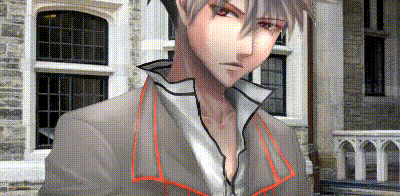{

GameObject temp = GameObject.Find("ui_selectbts");

Animator an = temp.GetComponent<Animator>();

an.SetInteger("a_num", 1);

}

{

GameObject temp = GameObject.Find("ui_selectbts");

Animator an = temp.GetComponent<Animator>();

an.SetInteger("a_num", 2);

}

{

GameObject temp = GameObject.Find("ui_selectbts");

temp.transform.Find("bt1/Text").gameObject.GetComponent<Text>().text = elm["text1"];

temp.transform.Find("bt2/Text").gameObject.GetComponent<Text>().text = elm["text2"];

Animator an = temp.GetComponent<Animator>();

an.SetInteger("a_num", 1);

}

{

GameObject temp = GameObject.Find("ui_selectbts");

temp.transform.Find("bt1/Text").gameObject.GetComponent<Text>().text = elm["text1"];

temp.transform.Find("bt2/Text").gameObject.GetComponent<Text>().text = elm["text2"];

//获取bt1的button

Button b1 = temp.transform.Find("bt1").gameObject.GetComponent<Button>();

//将上边所挂在的事件（如果有的话）全部删掉

b1.onClick.RemoveAllListeners();

//挂载新的事件

{

//点击了选择支按钮后就先淡出选择支按钮

//然后跳转到传入的target1的标签

jumpscript1(elm["target1"]);

});

b1 = temp.transform.Find("bt2").gameObject.GetComponent<Button>();

b1.onClick.RemoveAllListeners();

{

jumpscript1(elm["target2"]);

});

Animator an = temp.GetComponent<Animator>();

an.SetInteger("a_num", 1);

}

delegate ()

{

jumpscript1(elm["target2"]);

}

()

{

jumpscript1(elm["target2"]);

}

bool onscript()

{

if(scriptnum>=myscript.Length)

{

return false;

}

string line = myscript[scriptnum];

print(line);

scriptnum++;

if(line.Length==0)

{

//如果这行为空就跳过这行

return true;

}

else if(line.Substring(0,1)=="@")

{

//如果行首有@就作为一个function执行

Dictionary<string, string> elm = new Dictionary<string, string>();

string[] temparr = Regex.Split(line.Substring(1), " ", RegexOptions.IgnoreCase);

string macroname = temparr;

if (temparr.Length > 1)

{

for (int i = 1; i < temparr.Length; i++)

{

string[] temparr1 = Regex.Split(temparr[i], "=", RegexOptions.IgnoreCase);

elm[temparr1] = temparr1;

}

}

if (elm.Count > 0)

{

SendMessage(macroname,elm);

}

else

{

SendMessage(macroname);

}

return false;

}

else if(line.Substring(0, 1) == "*")

{

//如果行首是*就视为标签不做任何处理直接执行下一行

return true;

}

else

{

//如果行首没有@就把文字赋值给dtext等待对话框里显示

dtext = line;

return true;

}

}

void jumpscript(Dictionary<string, string> elm)

{

jumpscript1(elm["target"]);

}

void jumpscript1(string target)

{

if (target != "")

{

for (int i = 0; i < myscript.Length; i++)

{

string temp = myscript[i];

if (temp == target)

{

scriptnum = i;

startscript();

return;

}

}

}

//如果要求跳转到不存在的标签，那就跳转到第一行

scriptnum = 0;

startscript();

}

*t1

@jumpscript target=*c1

*t2

*c1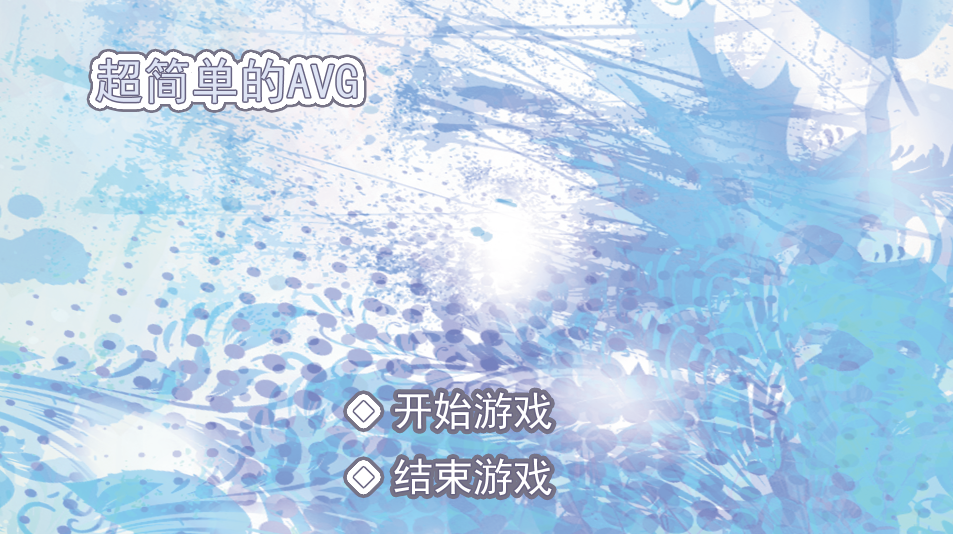{

GameObject temp = GameObject.Find("ui_opbts");

Animator an = temp.GetComponent<Animator>();

an.SetInteger("a_num", 1);

//给开始游戏按钮挂载事件

Button b1 = temp.transform.Find("bt1").gameObject.GetComponent<Button>();

b1.onClick.RemoveAllListeners();

{

//把开始界面按钮组淡出

//把背景淡出，执行下一行

});

//给结束游戏按钮挂载事件

b1 = temp.transform.Find("bt2").gameObject.GetComponent<Button>();

b1.onClick.RemoveAllListeners();

{

//结束游戏，游戏打包后才会生效，目前在测试模式下是无效的

Application.Quit();

});

}

{

GameObject temp = GameObject.Find("ui_opbts");

Animator an = temp.GetComponent<Animator>();

an.SetInteger("a_num", 2);

}

Application是UnityEngine的class，具体功能见https://docs.unity3d.com/ScriptReference/Application.html

*t1

@jumpscript target=*c1

*t2

*c1